﻿ 燃料相关组件棒流致振动可视化实验研究
«上一篇文章快速检索 高级检索

 哈尔滨工程大学学报2019, Vol. 40Issue (8): 1427-1432  DOI: 10.11990/jheu.2018040860

### 引用本文LIU Pengliang, LI Xin, LI Dongyang, et al. Visualization of flow-induced vibration of fuel-related assembling rod[J]. Journal of Harbin Engineering University, 2019, 40(8), 1427-1432. DOI: 10.11990/jheu.201804086.### 文章历史

1. 大连理工大学 运载工程与力学学部, 辽宁 大连 116024;
2. 中广核研究院有限公司, 广东 深圳 518031;
3. 哈尔滨工程大学 核科学与技术学院, 黑龙江 哈尔滨 115001

Visualization of flow-induced vibration of fuel-related assembling rod
LIU Pengliang 1,2, LI Xin 3, LI Dongyang 3, CHEN Biaosong 1, TAN Sichao 3
1. Dalian University of Technology, Dalian 116024, China;
2. China Nuclear Power Technology Research Institute Co., Ltd., Shenzhen 518031, China;
3. College of Nuclear Science and Technology, Harbin Engineering University, Harbin 150001, China
Abstract: The cantilever is a common structure in the reactor system. A turbulent excitation phenomenon occurs in the cantilever assembly rod during the axial outflow of the coolant in the washing-out phase, and this harmful vibration threatens the integrity of the associated structure. As yet, there is no effective means for measuring the flow-induced vibration characteristics of component rods with large length-to-diameter ratios and a very small annulus. To solve this problem, in this paper, we propose a visual measurement method that makes use of a high speed camera to study the vibration characteristics of the axial outflow of the cantilever beam. We obtained the dry mode parameters of the relevant component rods by applying external single-point excitation single-point pick-up. At the same time, we obtained the two-dimensional motion trajectory of the component rods by shooting two cameras simultaneously. The test results show that the vibration at the free end of the rods is more intense than at the fixed end. At low flow rates, the amplitude of the rod increases with increasing flow rate, and the amplitude is below 0.2 mm. At high flow rates, however, the rods come in contact with the wall surface at times, which decreases their amplitude.
Keywords: cantilever beam    flow-induced vibration    small gap    large aspect ratio    visual measurement    axial outflow    displacement response    dry mode

1 流致振动可视化实验系统和方法 1.1 实验系统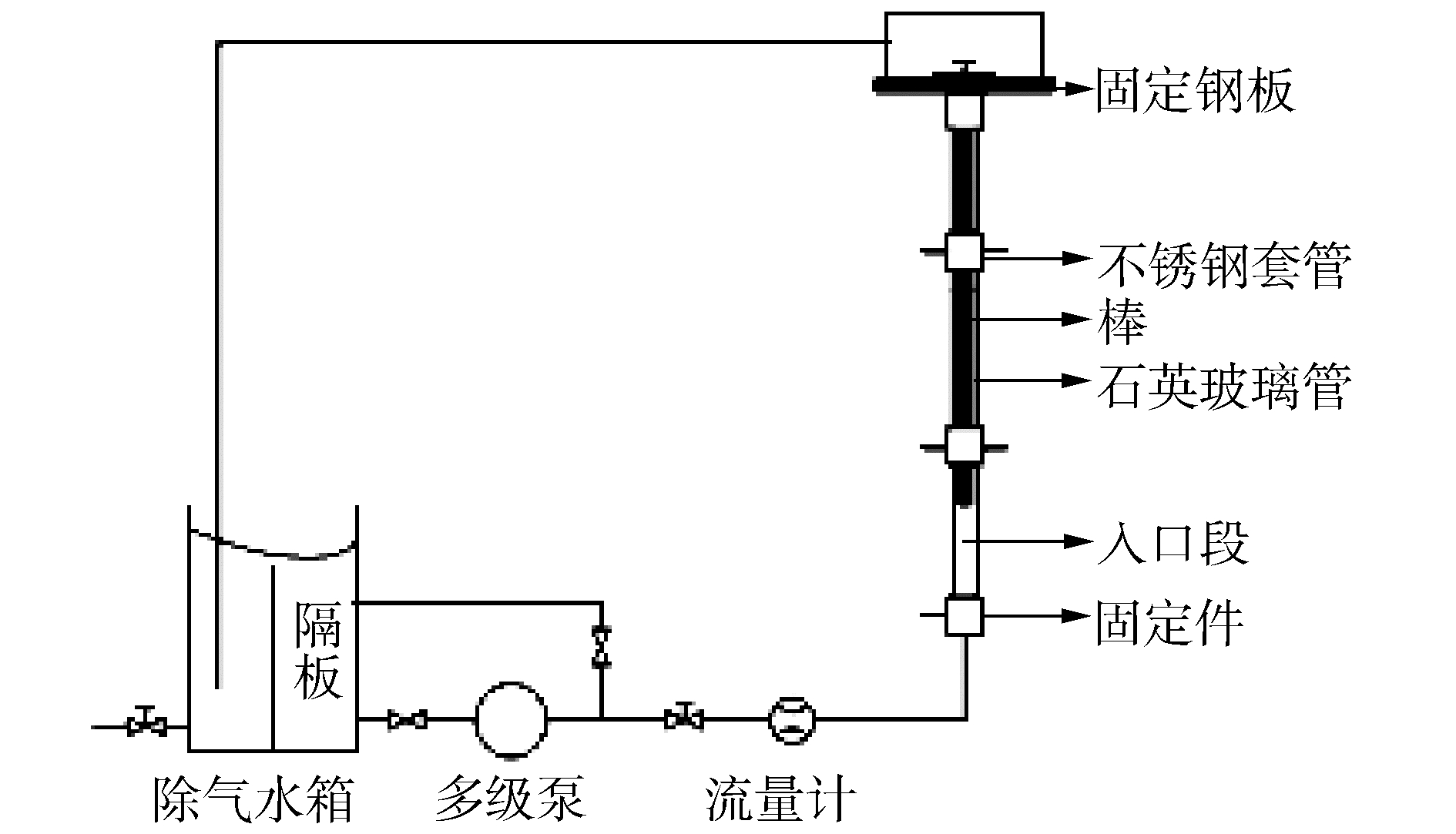Download: 图 1 实验流动系统 Fig. 1 Flow control system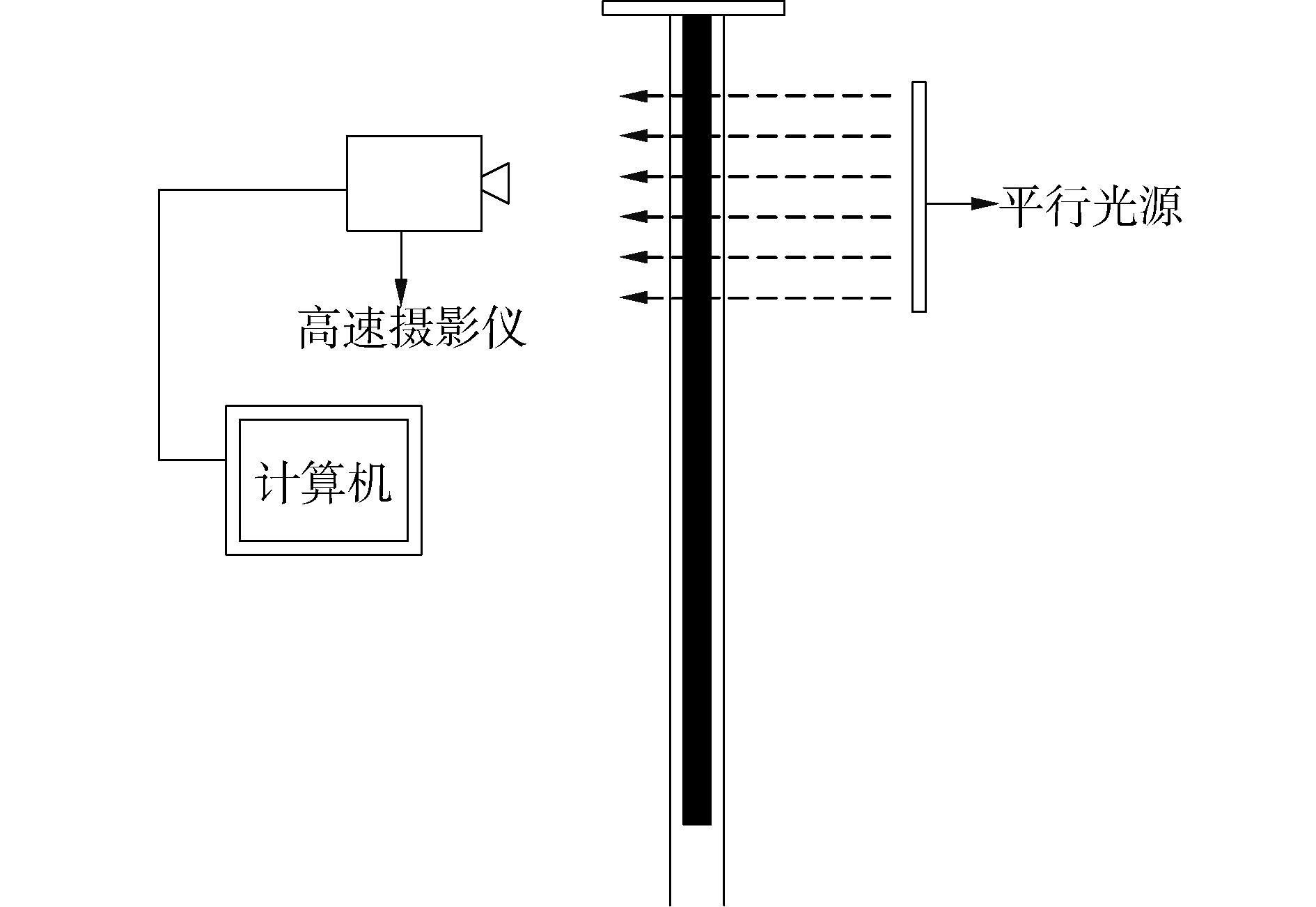Download: 图 2 实验可视化系统 Fig. 2 Visualized experiment system

1.2 实验方法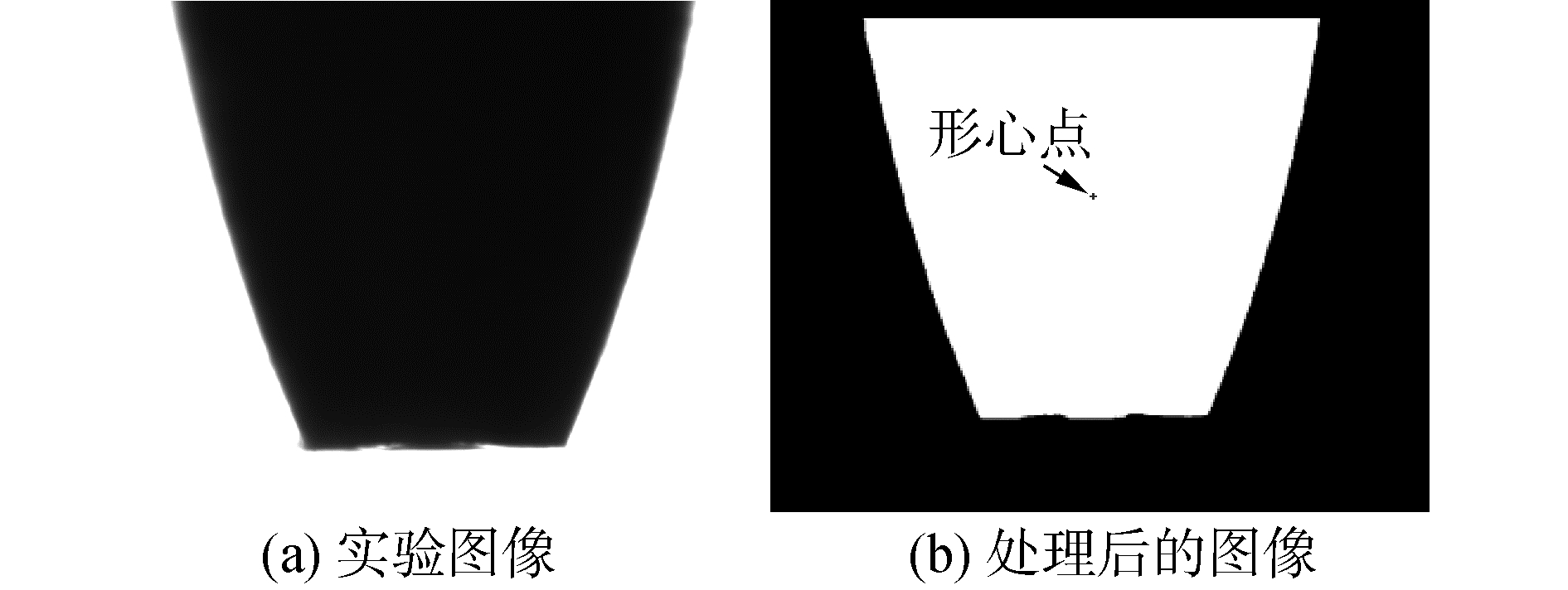Download: 图 3 图像处理及形心位置 Fig. 3 Image processing and centroid position
 $\left\{ \begin{array}{l} x = \sum\limits_{i = 1}^n {f\left( {x, y} \right)x} /\sum\limits_{i = 1}^n x \\ y = \sum\limits_{i = 1}^n {f\left( {x, y} \right)y} /\sum\limits_{i = 1}^n y \end{array} \right.$ (1)

2 模态实验结果

 $x = A{{\rm{e}}^{-\zeta {\omega _0}t}}\cos \left( {{\omega _d}t-\phi } \right)$ (2)

 $\left\{ \begin{array}{l} {\omega _1} = 1.875\;{104^2}{\left( {EI/\rho A{l^4}} \right)^{1/2}}\\ {\omega _2} = 4.694\;{091^2}{\left( {EI/\rho A{l^4}} \right)^{1/2}}\\ {\omega _3} = 7.854\;{757^2}{\left( {EI/\rho A{l^4}} \right)^{1/2}} \end{array} \right.$ (3)
3 流致振动实验结果及分析 3.1 实验工况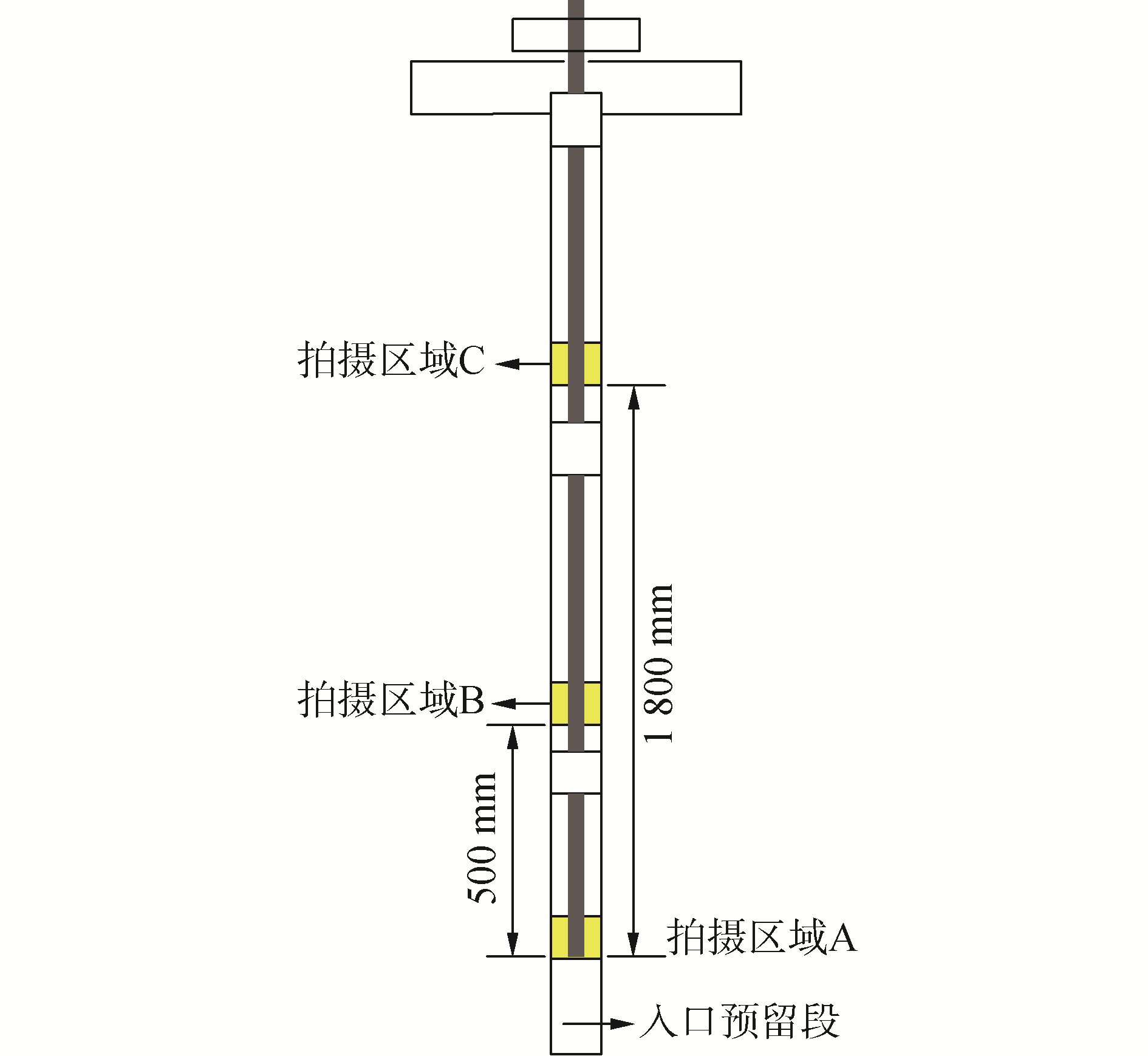Download: 图 4 测量区域 Fig. 4 Measurement region
3.2 一维位移响应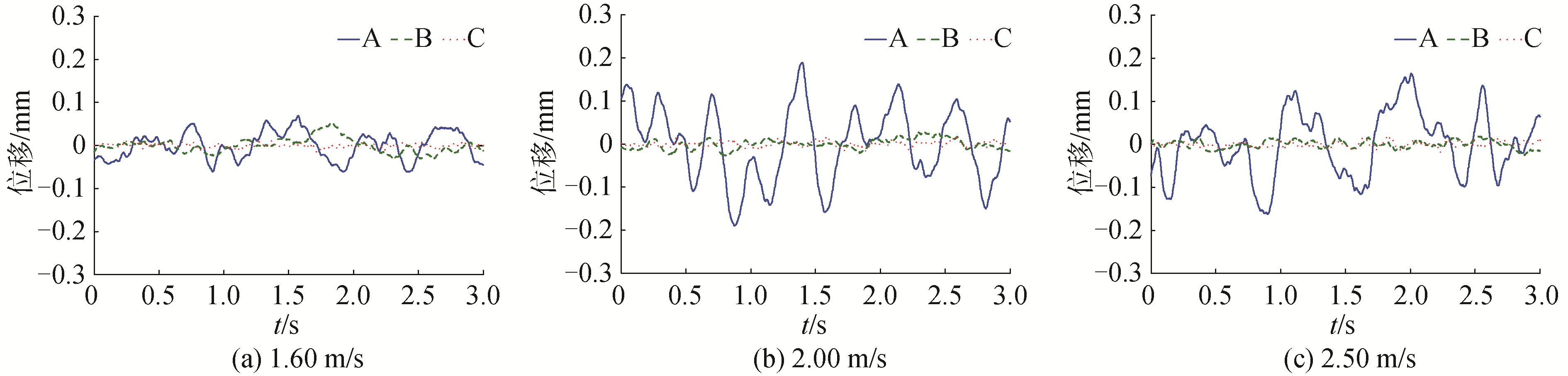Download: 图 5 不同流速下不同测量位置的振幅曲线 Fig. 5 Amplitude curves of under the various conditions and positions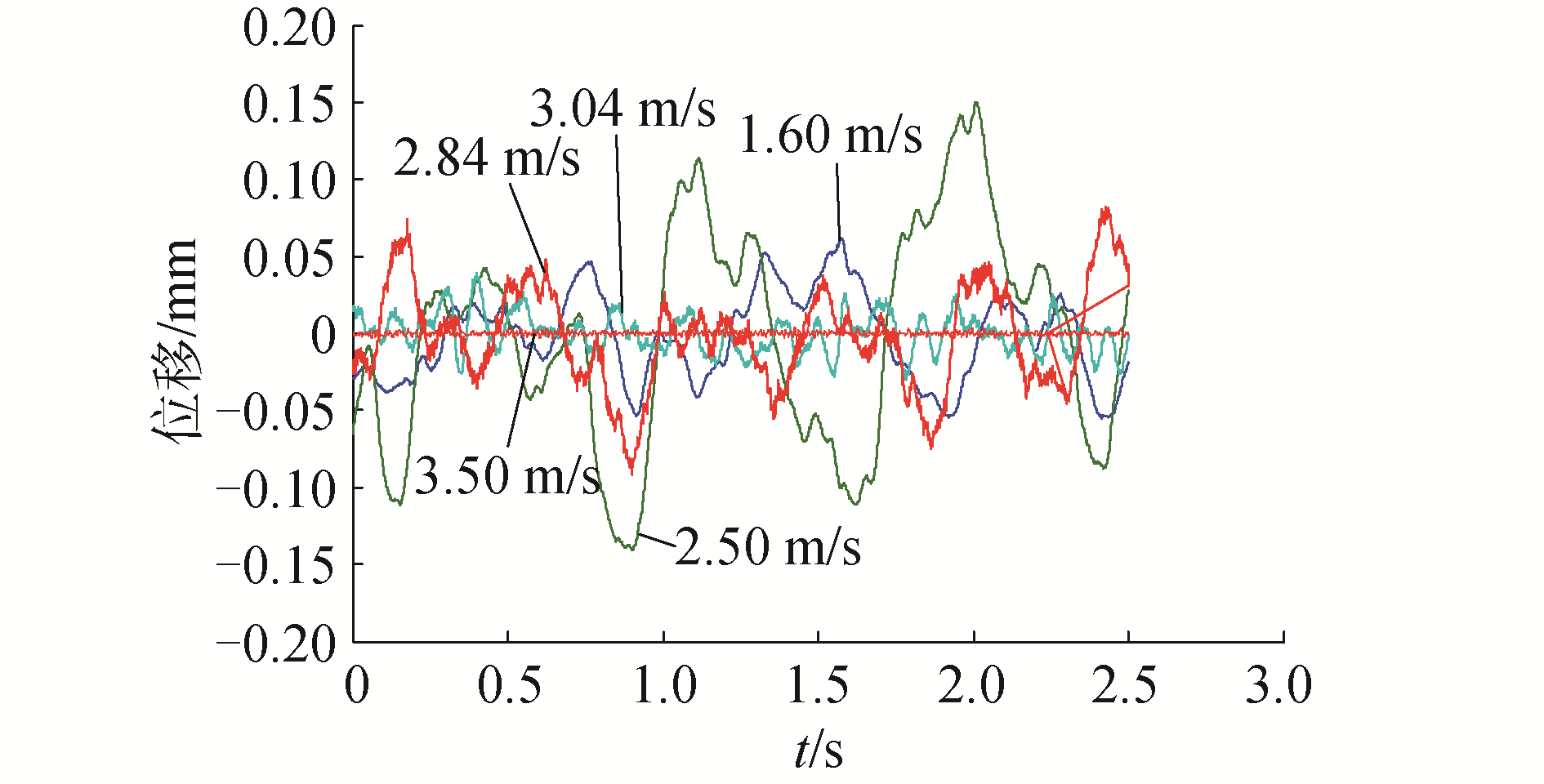Download: 图 6 不同流速下A位置振幅曲线 Fig. 6 Amplitude curves of under the various conditions

3.3 二维位移响应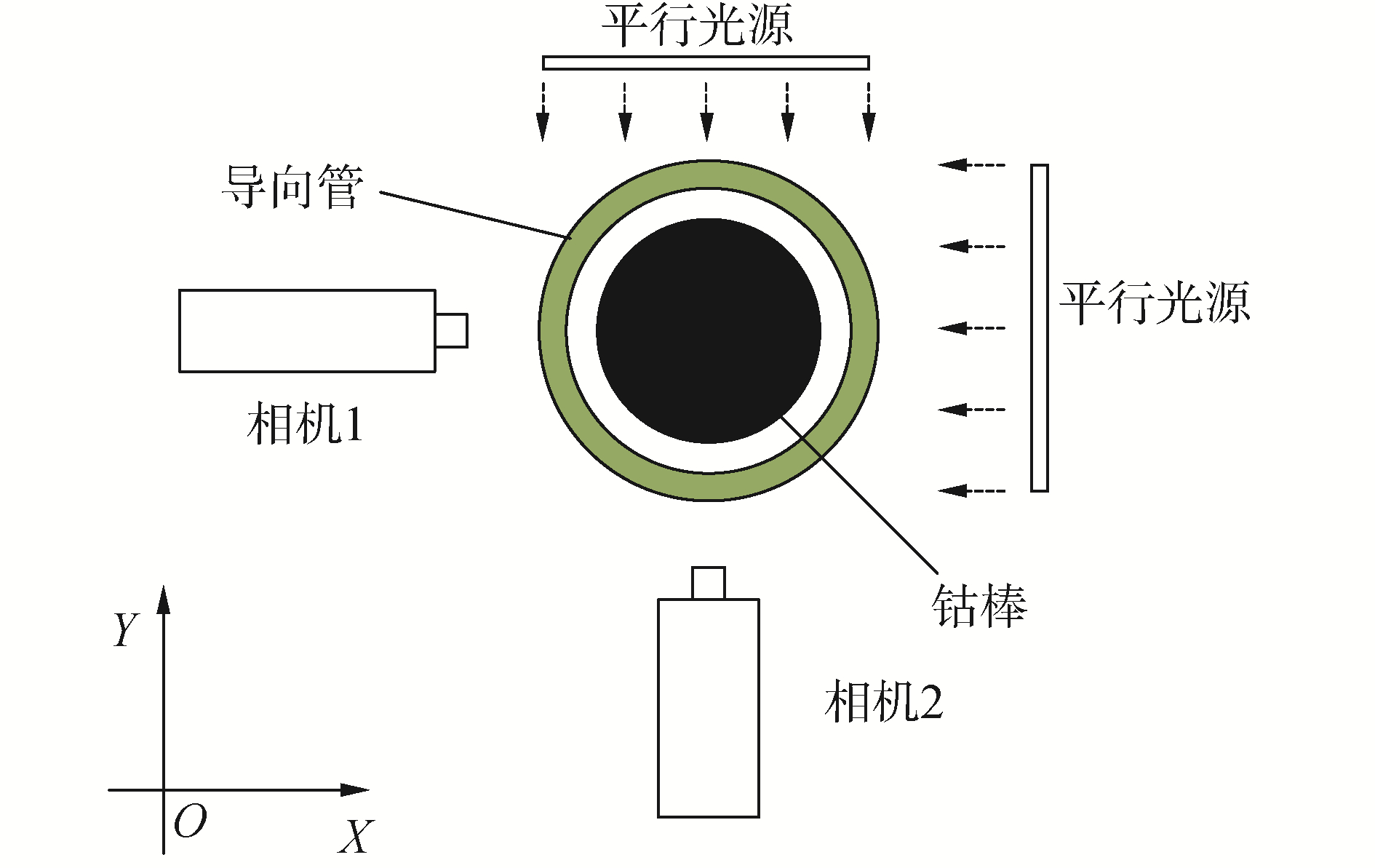Download: 图 7 二维振动实验拍摄示意 Fig. 7 Schematic of two-dimensional vibration measurement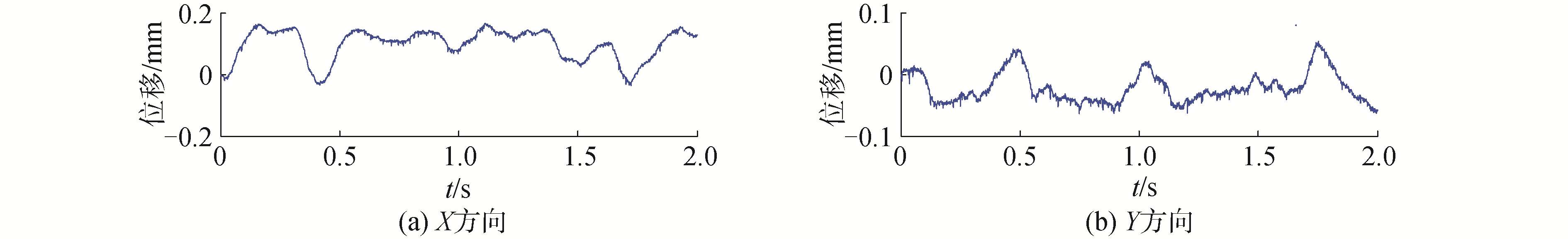Download: 图 8 组件棒在1.6 m/s时振动振幅曲线 Fig. 8 Amplitude curve on flow condition of 1.6 m/s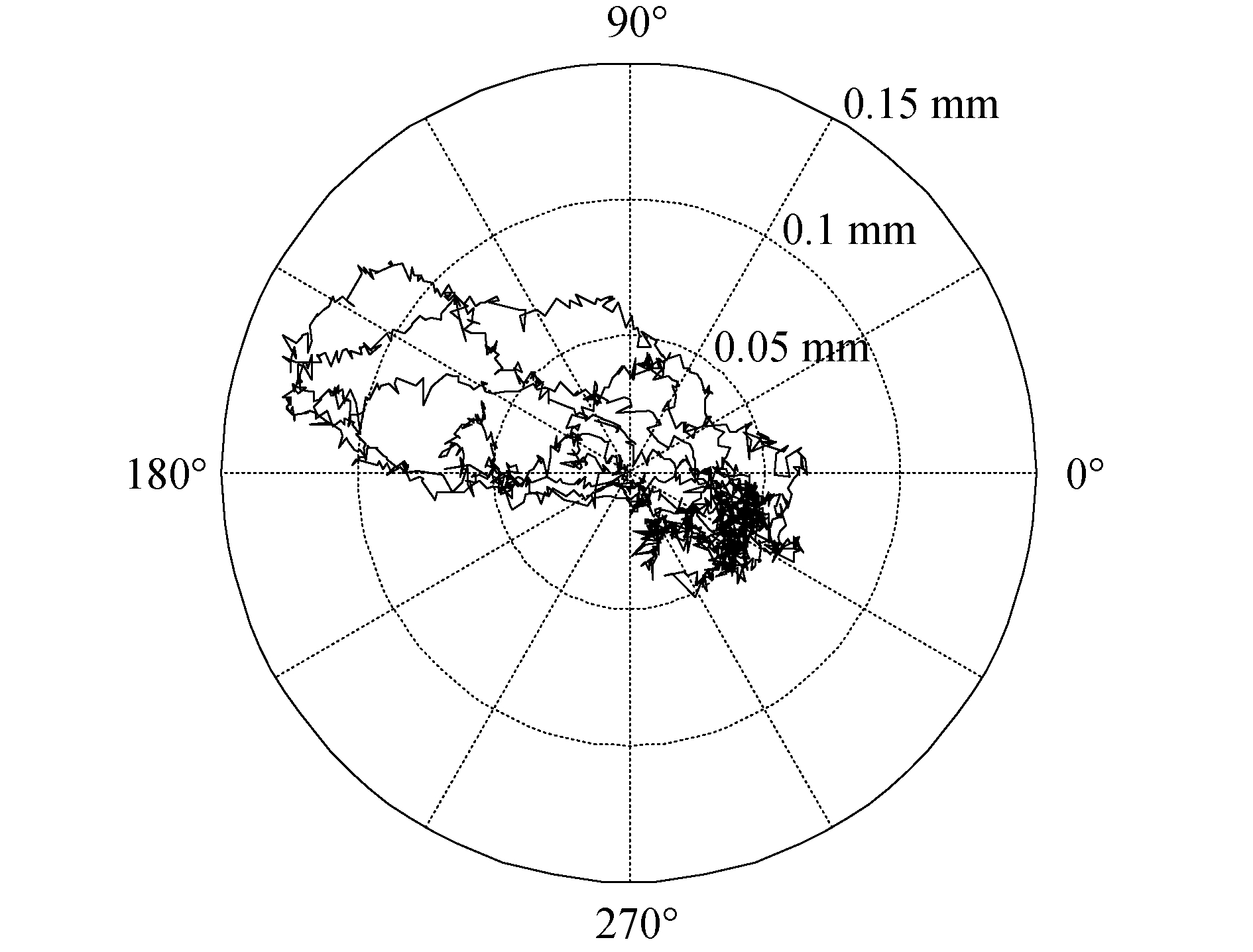Download: 图 9 相关组件棒在1.6 m/s时振动二维轨迹 Fig. 9 Schematic of trajectory on flow condition of 1.6 m/s
4 结论

1) 本文实现对流致振动的无干扰实时测量，获取振幅、频率等振动特性，测量精度高。利用模态分析证实该方法切实可行，并利用该方法对燃料相关组件棒流致振动特性进行研究。

2) 利用该方法对具有大长径比、极小环隙空间的导向管内燃料相关组件棒流致振动特性进行了实验研究，得到其在不同测量区域和流速下的一维振动特性：棒的自由端振动最剧烈，近固定端区域几无振动；低流速时，棒的振幅随着流速增大而增大，而高流速时，棒存在间歇接触壁面的情况，振幅呈减小趋势。

3) 通过2台相机拍摄，获得相关组件棒在二维平面内的二维振动轨迹和振动特性曲线，其轨迹呈现出振动的随机性。

  AIT ABDERRAHMANE H, PAIDOUSSIS M P, FAYED M, et al. Flapping dynamics of a flexible filament[J]. Physical review E, 2011, 84(6): 066604. (0)  王鹏.双圆柱体布局下的轴向流致振动实验研究[D].哈尔滨: 哈尔滨工业大学, 2017. WANG Peng. Axial flow-induced vibration of a twin cylinder configuration[D]. Harbin: Harbin Institute of Technology, 2017. (0)  PAÏDOUSSIS M P, PRICE S J, DE LANGRE E. Fluid-structure interactions:cross-flow-induced instabilities[M]. Cambridge: Cambridge University Press, 2011. (0)  CHEN S S. Flow-induced vibration of circular cylindrical structures[M]. Berlin: Springer-Verlag, 1987: 63. (0)  孙汉虹, 程平东, 缪鸿兴, 等. 第三代核电技术AP1000[M]. 北京: 中国电力出版社, 2010. (0)  邢景棠, 周盛, 崔尔杰. 流固耦合力学概述[J]. 力学进展, 1997, 27(1): 19-38. XING Jingtang, ZHOU Sheng, CUI Erjie. A survey on the fluid-solid interaction mechanics[J]. Advances in mechanics, 1997, 27(1): 19-38. DOI:10.3321/j.issn:1000-0992.1997.01.002 (0)  包日东, 闻邦椿. 水下悬跨输流管道流致振动响应研究[J]. 地震工程与工程振动, 2009, 29(3): 115-120. BAO Ridong, WEN Bangchun. Study on dynamic responses of submarine fluid conveying pipeline under eddy stimulation[J]. Earthquake engineering and engineering vibration, 2009, 29(3): 115-120. (0)  冯志鹏, 臧峰刚, 张毅雄, 等. 流体诱发传热管振动的流场特性分析[J]. 核动力工程, 2014, 35(2): 71-75. FENG Zhipeng, ZANG Fenggang, ZHANG Yixiong, et al. Flow characteristic analysis of flow induced heat exchanger tube vibration[J]. Nuclear power engineering, 2014, 35(2): 71-75. (0)  喻丹萍, 胡永陶. 秦山核电二期工程反应堆堆内构件模型流致振动试验研究[J]. 核动力工程, 2003, 24(S1): 109-113. YU Danping, HU Yongtao. Experimental study of flow-induced vibration of the reactor internal in Qinshan phase Ⅱ NPP project[J]. Nuclear power engineering, 2003, 24(S1): 109-113. (0)  蒋莉, 王建立, 孙成海, 等. 有界域轴向流动棒束流致振动附加质量力模型[J]. 原子能科学技术, 1999, 33(5): 431-435. JIANG Li, WANG Jianli, SUN Chenghai, et al. Mathematical models for fluid inertial forces added on the rod bundle vibrating in the bounded axial flow[J]. Atomic energy science and technology, 1999, 33(5): 431-435. (0)  KANG H S, MURIETHI N K, PETTIGREW M J. An experimental investigation on the narrow annular-flow-induced vibration instability of a tube[M]//KASSAB A J, BREBBIA C A, DIVO E A, et al. Fluid Structure Interaction VI. Southampton: WIT Press, 2011: 11-19. (0)  杨安良, 龚圣捷, 顾汉洋. 流致振动激光多普勒测振方法研究[J]. 测试技术学报, 2015, 29(2): 93-99. YANG Anliang, GONG Shengjie, GU Hanyang. An investigation of laser Doppler vibrometer measurement technique on flow-induced vibration[J]. Journal of test and measurement technology, 2015, 29(2): 93-99. (0)  刘军, 高建立, 穆桂脂, 等. 改进锤击法试验模态分析技术的研究[J]. 振动与冲击, 2009, 28(3): 174-177. LIU Jun, GAO Jianli, MU Guizhi, et al. An improved experimental modal analysis system with hammering method[J]. Journal of vibration and shock, 2009, 28(3): 174-177. DOI:10.3969/j.issn.1000-3835.2009.03.041 (0)  DE PAUW B, WEIJTJENS W, VANLANDUIT S, et al. Operational modal analysis of flow-induced vibration of nuclear fuel rods in a turbulent axial flow[J]. Nuclear engineering and design, 2015, 284: 19-26. (0)  吴毓熙. 应用弹性力学[M]. 上海: 同济大学出版社, 1989. (0)